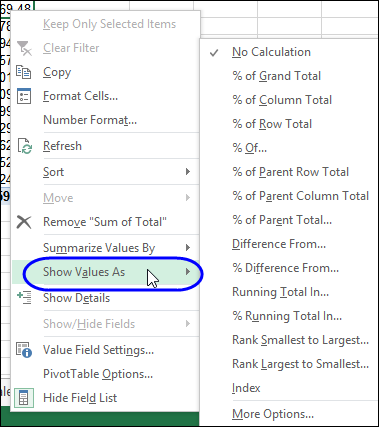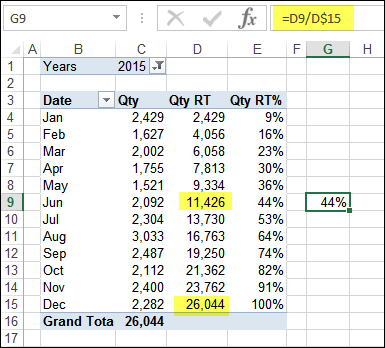# Pivot Table Running Total Percent

At the beginning of every month, I download the previous month's statistics for my web site, to see which pages and files were the most popular. After the data is imported to Excel, I use pivot tables to get a quick overview of the activity.

If a page is getting lots of hits, I might add a new section to it, to make the content even better. And, seeing which sample files get downloaded most frequently gives me ideas for creating new examples.

I'm sure you do something similar for your month end, to see how things are going. And the raw statistics are interesting, but with a pivot table (or 12), you can dig even deeper into the results.### Pivot Table "Show Values As"

When you add a number field to a pivot table, it usually shows up in the Values area, summarized by Sum or Count. There are lots of other options though, such as Average, Minimum and Maximum.

Also, there are many ways to view those Sums or Counts. Just right-click a Value field, and click "Show Values As", to see what's available, such as % of Column Total, or Running Total In.### % Running Total In

One of my favourite options is the % Running Total calculation, which shows the current running total amount, divided by the grand total. This option was added in Excel 2010.

In the pivot table shown below, there are three Value fields in the pivot table, showing the Sum of Qty sold in each month.

• In column C, the sum is shown, with no calculation. This is the number of units sold each month.
• In column D, the sum is shown, as a Running Total for Date. This is the total units sold, up to and including that month.
• In column E, the sum is shown, as % Running Total for Date (new in Excel 2010). This is the total units sold, up to and including that month, divided by the grand total of units sold.

By June, a running total of 11426 units have been sold, which is 44% of the overall total units sold. I verified that, buy using a formula in cell G9, to divide the June running total, by the grand total.

So, sales went better in the second half of the year – the remaining 56% of the sales occurred then.### Video: Running Total Percent

Watch this short video to see how to set up the pivot table, add a running total percent, change the row field and adjust the running total percent.

00:00    Introduction
00:19    Create the Pivot Table
01:09    Add Fields to Pivot Table
01:36    Sort by Sales Amount
01:55    Add Running Total Percent
02:44    Read the Running Total Per
03:23    Change the Row Field
04:13    Fix the Running Total Percent
04:31    Running Total Percent by Month
04:59    Get the Sample File